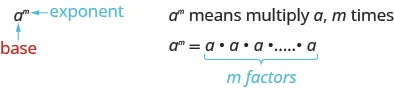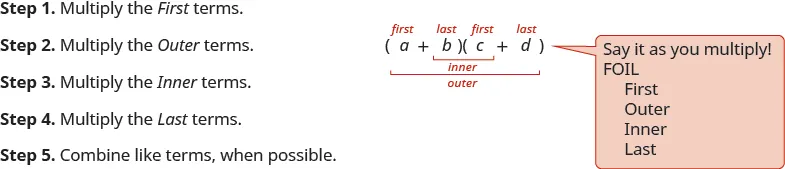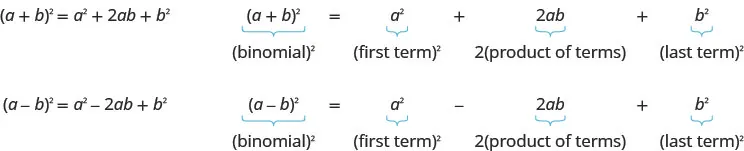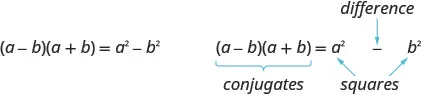Intermediate Algebra 2e

# Key Concepts

### Key Concepts

• Monomial
• A monomial is an algebraic expression with one term.
• A monomial in one variable is a term of the form $axm,axm,$ where a is a constant and m is a whole number.
• Polynomials
• Polynomial—A monomial, or two or more algebraic terms combined by addition or subtraction is a polynomial.
• monomial —A polynomial with exactly one term is called a monomial.
• binomial — A polynomial with exactly two terms is called a binomial.
• trinomial —A polynomial with exactly three terms is called a trinomial.
• Degree of a Polynomial
• The degree of a term is the sum of the exponents of its variables.
• The degree of a constant is 0.
• The degree of a polynomial is the highest degree of all its terms.

#### 5.2Properties of Exponents and Scientific Notation

• Exponential NotationThis is read a to the $mthmth$ power.
In the expression $amam$, the exponent m tells us how many times we use the base a as a factor.
• Product Property for Exponents
If a is a real number and m and n are integers, then
$am·an=am+nam·an=am+n$

To multiply with like bases, add the exponents.
• Quotient Property for Exponents
If $aa$ is a real number, $a≠0,a≠0,$ and m and n are integers, then
$aman=am−n,m>nandaman=1an−m,n>maman=am−n,m>nandaman=1an−m,n>m$
• Zero Exponent
• If a is a non-zero number, then $a0=1.a0=1.$
• If a is a non-zero number, then a to the power of zero equals 1.
• Any non-zero number raised to the zero power is 1.
• Negative Exponent
• If n is an integer and $a≠0,a≠0,$ then $a−n=1ana−n=1an$ or $1a−n=an.1a−n=an.$
• Quotient to a Negative Exponent Property
If $a,ba,b$ are real numbers, $a≠0,b≠0a≠0,b≠0$ and $nn$ is an integer, then
$(ab)−n=(ba)n(ab)−n=(ba)n$
• Power Property for Exponents
If $aa$ is a real number and $m,nm,n$ are integers, then
$(am)n=am·n(am)n=am·n$

To raise a power to a power, multiply the exponents.
• Product to a Power Property for Exponents
If a and b are real numbers and m is a whole number, then
$(ab)m=ambm(ab)m=ambm$

To raise a product to a power, raise each factor to that power.
• Quotient to a Power Property for Exponents
If $aa$ and are real numbers, $b≠0,b≠0,$ and $mm$ is an integer, then
$(ab)m=ambm(ab)m=ambm$

To raise a fraction to a power, raise the numerator and denominator to that power.
• Summary of Exponent Properties
If a and b are real numbers, and m and n are integers, then

Property Description
Product Property $am·an=am+nam·an=am+n$
Power Property $(am)n=am·n(am)n=am·n$
Product to a Power $(ab)n=anbn(ab)n=anbn$
Quotient Property $aman=am−n,a≠0aman=am−n,a≠0$
Zero Exponent Property $a0=1,a≠0a0=1,a≠0$
Quotient to a Power Property: $(ab)m=ambm,b≠0(ab)m=ambm,b≠0$
Properties of Negative Exponents $a−n=1ana−n=1an$ and $1a−n=an1a−n=an$
Quotient to a Negative Exponent $(ab)−n=(ba)n(ab)−n=(ba)n$
• Scientific Notation
A number is expressed in scientific notation when it is of the form
$a×10nwhere1≤a<10andnis an integer.a×10nwhere1≤a<10andnis an integer.$
• How to convert a decimal to scientific notation.
1. Step 1. Move the decimal point so that the first factor is greater than or equal to 1 but less than 10.
2. Step 2. Count the number of decimal places, $n,n,$ that the decimal point was moved.
3. Step 3.
Write the number as a product with a power of 10. If the original number is.
• greater than 1, the power of 10 will be $10n.10n.$
• between 0 and 1, the power of 10 will be $10−n.10−n.$
4. Step 4. Check.
• How to convert scientific notation to decimal form.
1. Step 1. Determine the exponent, $n,n,$ on the factor 10.
2. Step 2.
Move the decimal $nn$ places, adding zeros if needed.
• If the exponent is positive, move the decimal point $nn$ places to the right.
• If the exponent is negative, move the decimal point $|n||n|$ places to the left.
3. Step 3. Check.

#### 5.3Multiply Polynomials

• How to use the FOIL method to multiply two binomials.• Multiplying Two Binomials: To multiply binomials, use the:
• Distributive Property
• FOIL Method
• Multiplying a Polynomial by a Polynomial: To multiply a trinomial by a binomial, use the:
• Distributive Property
• Vertical Method
• Binomial Squares Pattern
If a and b are real numbers,• Product of Conjugates Pattern
If $a,ba,b$ are real numbersThe product is called a difference of squares.
To multiply conjugates, square the first term, square the last term, write it as a difference of squares.
• Comparing the Special Product Patterns
Binomial Squares Product of Conjugates
$(a+b)2=a2+2ab+b2(a+b)2=a2+2ab+b2$ $(a−b)(a+b)=a2−b2(a−b)(a+b)=a2−b2$
$(a−b)2=a2−2ab+b2(a−b)2=a2−2ab+b2$
•  Squaring a binomial •  Multiplying conjugates
•  Product is a trinomial •  Product is a binomial.
•  Inner and outer terms with FOIL are the same. •  Inner and outer terms with FOIL are opposites.
•  Middle term is double the product of the terms •  There is no middle term.
• Multiplication of Polynomial Functions:
• For functions $f(x)f(x)$ and $g(x),g(x),$
$(f·g)(x)=f(x)·g(x)(f·g)(x)=f(x)·g(x)$

#### 5.4Dividing Polynomials

• Division of a Polynomial by a Monomial
• To divide a polynomial by a monomial, divide each term of the polynomial by the monomial.
• Division of Polynomial Functions
• For functions $f(x)f(x)$ and $g(x),g(x),$ where $g(x)≠0,g(x)≠0,$
$(fg)(x)=f(x)g(x)(fg)(x)=f(x)g(x)$
• Remainder Theorem
• If the polynomial function $f(x)f(x)$ is divided by $x−c,x−c,$ then the remainder is $f(c).f(c).$
• Factor Theorem: For any polynomial function $f(x),f(x),$
• if $x−cx−c$ is a factor of $f(x),f(x),$ then $f(c)=0f(c)=0$
• if $f(c)=0,f(c)=0,$ then $x−cx−c$ is a factor of $f(x)f(x)$
Order a print copy

As an Amazon Associate we earn from qualifying purchases.